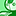# QlikView App Dev

Discussion Board for collaboration related to QlikView App Development.

Announcements
QlikWorld 2023, a live, in-person thrill ride. Save \$300 before February 6: REGISTER NOW!
cancel
Showing results for
Did you mean:Creator II

## Converting into set analysis

Hi,

I want to covert following expression into set analysis and add filters([prod date] and [manager name]) like below expression given as an eg ) to the expression below

=interval(div(sum(aggr(avg(distinct(TI_AUX)),agent_ID,DT_time_key)),3600)

&':'&(div(sum(aggr(avg(dstinct(TI_AUX)),agent_ID,DT_time_key)),60)-div(sum(aggr(avg(dstinct(TI_AUX)),agent_ID,DT_time_key)),3600)*60,'HH:mm')

I have already written an expression for some another requirement using set analysis. In a similar manner I want to convert the above expression with filters

eg-

=num(sum({1<[sujct]={'vol'},[prod date]=p[prod date],[manager name]=p[manager name])>[volume count]),'#,##0')

2 RepliesSpecialist II

Hi,

I think generally you will need your set at every point you aggregate (so for each sum/avg in the expression). Similar to the reply here;

Solved: Aggr Nested in Set Analysis - Qlik Community - 216128

Cheers,

Chris.Contributor III
@myccpay wrote:

Hi,

I want to covert following expression into set analysis and add filters([prod date] and [manager name]) like below expression given as an eg ) to the expression below

=interval(div(sum(aggr(avg(distinct(TI_AUX)),agent_ID,DT_time_key)),3600)

&':'&(div(sum(aggr(avg(dstinct(TI_AUX)),agent_ID,DT_time_key)),60)-div(sum(aggr(avg(dstinct(TI_AUX)),agent_ID,DT_time_key)),3600)*60,'HH:mm')

I have already written an expression for some another requirement using set analysis. In a similar manner I want to convert the above expression with filters

eg-

=num(sum({1<[sujct]={'vol'},[prod date]=p[prod date],[manager name]=p[manager name])>[volume count]),'#,##0')